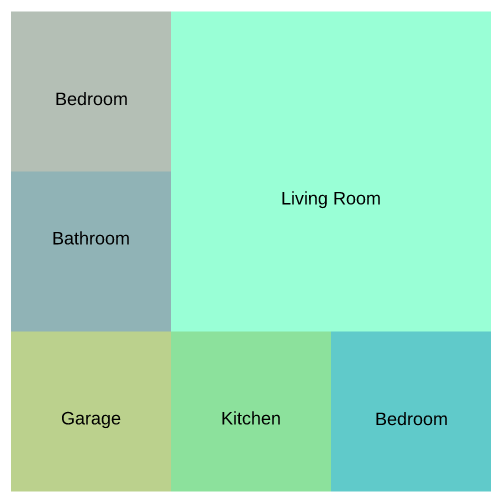# Covering a square with N disjoint squares

I could clearly fit in 4 squares together to make a square. Or, I could fit in 6 squares into a square as well:But this is not possible with 3 squares (or so I think!)

How does this generalize? How can we decide for which N such a covering is possible?

For any even number $N=2m>2$, you can tile using $2m-1$ squares of size $1\times 1$ along two sides of an $(m-1)\times(m-1)$ square. This gives solutions for $N=4, 6, 8, 10,\ldots$.
Finally, if you have a tiling with $n$ squares, you can make one with $n+3$ squares by cutting one of the tiles into 4 smaller ones. Just apply this once to the above solution and you have $N=7, 9, 11, \ldots$ solved.
Thus, there are tilings for all $N$ except 2, 3, and 5.